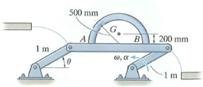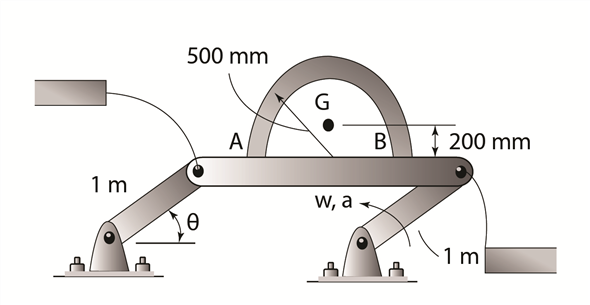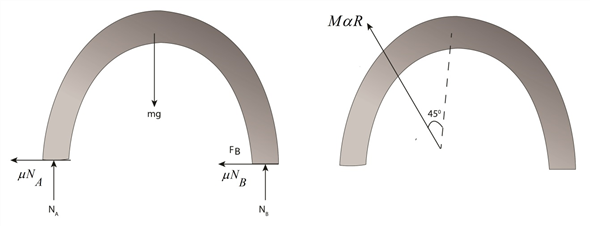Problem

# The arched pipe has a mass of 80 kg and rests on the surface of the platform for which the...

The arched pipe has a mass of 80 kg and rests on the surface of the platform for which the coefficient of static friction is μs = 0.3. Determine the greatest angular acceleration α of the platform, starting from rest when θ = 45°, without causing the pipe to slip on the platform.#### Step-by-Step Solution

Solution 1

Schematic diagram of the pipe is shown,Free body diagram of the pipe is as shown,Consider the free body diagram of the arched pipe, write the equation of equilibrium in horizontal direction:

$$\mu_{s} N_{A}+\mu_{s} N_{B}=m R \alpha \sin \theta$$

Here, $$N_{A}$$ and $$N_{B}$$ are the normal reactions at $$A$$ and $$B$$ respectively, $$\mu_{s}$$ is the coefficient of static friction, $$m$$ mass of the arched beam, a is angular acceleration and $$\theta$$ is angle made by the arm with horizontal axis.

Substitute, $$0.3$$ for $$\mu_{s}, 80 \mathrm{~kg}$$ for $$m, 1$$ for $$R$$ and $$45^{\circ}$$ for $$\theta$$.

$$0.3 N_{A}+0.3 N_{B}=80 \times 1(\alpha) \sin 45^{\circ}$$

$$0.3 N_{A}+0.3 N_{B}-56.56(\alpha)=0$$

Divide both sides with $$0.3 .$$

$$N_{A}+N_{B}-188.54(\alpha)=0 \ldots \ldots(1)$$

Write the equation of equilibrium in vertical-direction

$$N_{A}+N_{B}-m g=m R \alpha \cos \theta$$

Substitute, $$80 \mathrm{~kg}$$ for $$m, 9.81 \mathrm{~m} / \mathrm{s}^{2}$$ for $$g, 1$$ for $$\mathrm{R}$$ and $$45^{\circ}$$ for $$\theta$$ in equation (2)

$$N_{A}+N_{B}-80 \times 1 \times 9.81=80 \times 1(\alpha) \cos 45^{\circ}$$

$$N_{A}+N_{B}-56.56(\alpha)=784.8 \ldots \ldots .(2)$$

Subtract equation (2) from equation (1).

\begin{aligned}-188.54(\alpha)-(-56.56(\alpha)) &=-784.8 \\-188.54(\alpha)+56.56(\alpha) &=-784.8 \\ \alpha &=5.946 \mathrm{~m} / \mathrm{s}^{2} \end{aligned}

Therefore, the angular acceleration is, $$5.946 \frac{\mathrm{rad}}{\mathrm{s}^{2}}$$